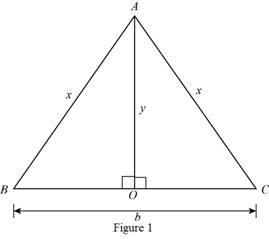# The function that models the area A in terms of length of its base b of an isosceles triangle.### Precalculus: Mathematics for Calcu...

6th Edition
Stewart + 5 others
Publisher: Cengage Learning
ISBN: 9780840068071### Precalculus: Mathematics for Calcu...

6th Edition
Stewart + 5 others
Publisher: Cengage Learning
ISBN: 9780840068071

#### Solutions

Chapter 2, Problem 15P
To determine

## To find: The function that models the area A in terms of length of its base b of an isosceles triangle.

Expert Solution

The function that model the area of isosceles triangle is A(b)=b4b.

### Explanation of Solution

The isosceles triangle is shown below.Area of triangle is given by,

A=12(baseheight)=12(by) (1)

As shown in Figure 1 height is y and base is b.

Perimeter P of triangle shown in Figure 1 is,

P=x+x+b=2x+b

Substitute P=8 in above equation to get x in terms of b.

8=2x+b8b=2x+bb8b=2xx=8b2

Apply Pythagorean Theorem in triangle ABO

(AB)2=(OB)2+(OA)2

Substitute AB=x, OB=b2 and AO=y in above equation.

(x)2=(b2)2+(y)2

Substitute x=8b2 in above equation and get y in terms of b.

(8b2)2=(b2)2+(y)2164b+b24=b24+y2164b+b24b24=b24+y2b24164b=y2

Take square root of above equation.

164b=y24b=y

Substitute 24b for y in equation (1)

A=12(b24b)A=b4b

Thus, the function that model the area of isosceles triangle is A(b)=b4b.

### Have a homework question?

Subscribe to bartleby learn! Ask subject matter experts 30 homework questions each month. Plus, you’ll have access to millions of step-by-step textbook answers!